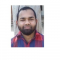## How to create a vertical line in a time series plot in base R?Nizamuddin Siddiqui

Updated on 23-Nov-2021 05:42:07

To create a time series plot, we can use simply apply plot function on time series object and if we want to create a vertical line on that plot then abline function will be used with v argument.For example, if we have a time series object called T and we ... Read More

## How to change the order of a matrix in increasing order based on a single column?Nizamuddin Siddiqui

Updated on 23-Nov-2021 05:40:26

To change the order of a matrix in increasing order based on a single column in R, we can use order function after subsetting a particular column.For example, if we have a matrix called M and we want to change the order of M in increasing order based on first ... Read More

## How to convert matrices stored in a list into vectors in R?Nizamuddin Siddiqui

Updated on 23-Nov-2021 05:34:15

A list may contain vectors, data frames, matrices, lists etc. If a list contains matrices and we want to convert those matrices into vectors then lapply function can be used along with as.vector function.For example, if we have a list called LIST that contains matrices then we can convert those ... Read More

## How to create a column with ratio of two columns in R?Nizamuddin Siddiqui

Updated on 23-Nov-2021 05:27:06

To create a new column with ratio of two columns in an R data frame, we can use division sign.For example, if we have a data frame called df that contains two columns say X and Y and we want to create a new column with ratio of X and ... Read More

## How to find the number of times a variable changes its sign in an R data frame column?Nizamuddin Siddiqui

Updated on 23-Nov-2021 05:22:20

To find the number of times a variable changes its sign in an R data frame column, we can use sign function with diff and sum function.For example, if we have a data frame called df that contains a column say C then, we can find the number of times ... Read More

## How to change the color of line in xyplot in R?Nizamuddin Siddiqui

Updated on 22-Nov-2021 08:22:10

To change the color of line in xyplot, we can use col argument.For example, if we have two vectors say X and Y and we want to create a red colored xyplot between X and Y then we can use the following command −xyplot(x~y, type="l", col="red")Check out the below example ... Read More

## How to fill the outliers with different color in base R boxplot?Nizamuddin Siddiqui

Updated on 22-Nov-2021 08:21:09

To fill the outliers in boxplot with different color in base R, we can use outpch argument for the shape and outbg argument for colors.For example, if we have a vector called X that contains some outliers then we can create a boxplot of X with different color outliers by ... Read More

## How to display outliers in boxplot with different shape in base R?Nizamuddin Siddiqui

Updated on 22-Nov-2021 08:19:41

To display outliers in boxplot with different shape in base R, we can use outpch argument in boxplot.For example, if we have a vector called X that contains some outliers then we can create a boxplot of X with different shape of outliers by using the below given command −boxplot(X, ... Read More

## How to change the color of box of boxplot in base R?Nizamuddin Siddiqui

Updated on 22-Nov-2021 08:18:04

To change the color of box of boxplot in base R, we can use col argument inside boxplot function.For example, if we have a vector called V and we want to create a boxplot of V without red colored box then we can use the following command −boxplot(x, col="red")ExampleTo change ... Read More

## How to change the color of outliers in base R boxplot?Nizamuddin Siddiqui

Updated on 22-Nov-2021 08:16:26

To change the color of outliers in base R boxplot, we can use outcol argument in boxplot function.For example, if we have a vector called X that contains some outliers then we can create a boxplot of X with blue color outliers by using the below given command −boxplot(X, outcol="red")ExampleTo ... Read More